Wednesday, January 22, 2014

Mathematical Fingerprint of the Universe: Understand the power of 3, 9, 6 and Vortex Mathematics

Guthrie delivers another powerful discourse on numbers, spiral energy expression and the Roden Coil. This post goes along well with Light From Sound? Sonoluminescence creating the Heat of a Sun via Implosion - Understanding Over Unity Physics. Numerology has been around for thousands of years, and it is obvious that the ancient jewish language is deeply coded with numerological meanings.

Guthrie does a great job of explaining these complex concepts in a simple and associateable way.
- Justin

Source - Energy In Motion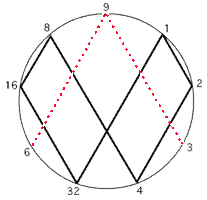Marko Rodin spent his life attempting to discover the natural mathematical flow of the universe. He had a distinct understanding of the underlying patterns that are the foundation of the reality we experience. He knew that numbers were not quantitative, rather QUALITATIVE. That they are not just a tally system for the covetous king to count his coin, but ALIVE and speaking, conveying to us the answers of the universe.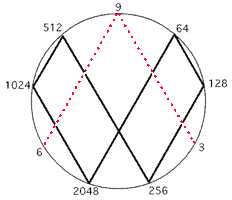Our journey begins with the number 9. In numerology all numbers above 9 are broken down until they are in base number form. This is done simply by adding the digits of larger numbers to eachother. For example: 42 = 4 + 2 = 6. There are a number of significant aspects to the base set of numbers, but for now we will focus on the numerical relationships. Marko Rodin knew of this adding principle and thought to explore it's geometrical relationship. He drew a circle with the numbers one through nine dispersed clockwise around it.

He began to connect the points using doubling. 1-2-4-8-16(7)-32(5).... He realized that no matter how many times he doubled the numbers in the sequence could always be broken down to the initial one through nine sequence.

The number is on the left, horizontal addition result is on the right. Notice how the 1,2,4,8,7,5 number pattern repeats indefinitely.
1 = 1
2 = 2
4 = 4
8 = 8
16 = 7
32 = 5
64 = 1
128 = 2
256 = 4
512 = 8
1,024 = 7
2,048 = 5
4,096 = 1
8,192 = 2
16,384 = 4
32,768 = 8
65,536 = 7
131,072 = 5
262,144 = 1
524,288 = 2
1,048,576 = 4
2,097,152 = 8
4,194,304 = 7
8,388,608 = 5
16,777,216 = 1
33,554,432 = 2
67,108,864 = 4
134,217,728 = 8
268,435,456 = 7
536,870,912 = 5
1,073,741,824 = 1
2,147,483,648 = 2
4,294,967,296 = 4
8,589,934,592 = 8

At this point you may be asking yourself, "what does this have to do with energy, are there any real applications for this sequence?" These numerical patterns link together very neatly to demonstrate how energy flows. The number system One through 9 is not man made but rather created by the natural manner in which energy flows. After 20 years of collaborating with engineers and scientists he found that the 1-2-4-8-7-5 sequence was a doubling circuit for an incredibly efficient electrical coil.
Below is a brief discourse on the Rodin Coil and it's energy harvesting properties.

The Fingerprint of GOD.

There was still one more very important number pattern to be realized. On the MATHEMATICAL FINGER PRINT OF GOD notice how the 3, 9, and 6 is in red and does not connect at the base. That is because it is a vector. The 1,2,4,8,7,5 is the third dimension while the oscillation between the 3 and 6 demonstrates the fourth dimension, which is the higher dimensional magnetic field of an electrical coil. The 3, 9, and 6 always occur together with the 9 as the control. In fact, the Yin/Yang is not a duality but rather a trinary. This is because the 3 and 6 represent each side of the Yin/Yang and the 9 is the "S" curve between them. Everything is based on thirds. We think that the universe is based on dualities because we see the effects not the cause.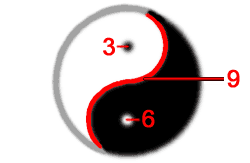When I say the oscillation between the 3 and 6 what I mean is that 3 doubled is 6 and 6 doubled is 12, but 1+2=3, and then 12 doubled is 24, but 2+4=6 and 24 doubled is 48, but 4+8=12 and 1+2=3. So the 3 and 6 go back and forth in their own separate dimension. This creates the other important pattern of (3,9,6,6,9,3,3,9).

Now lay out the number patterns on the 2D grid. Place the 3,9,6,6,9,3,3,9 number pattern in the center of the 1,2,4,8,7,5 number pattern going forward and the 1,2,4,8,7,5 number pattern going backwards this continuation creates an amazingly perfect number map. TheMATHEMATICAL FINGER PRINT OF GOD is a legend or blueprint for the torus and can be visualized in a 2D form below.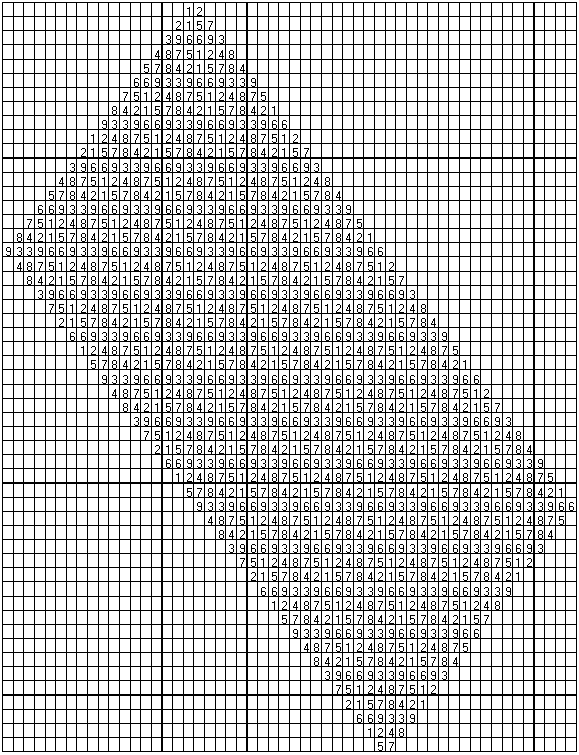The 2D number map forms the 3D skin of the torus and thus demonstrates the concept of the winding of the coil.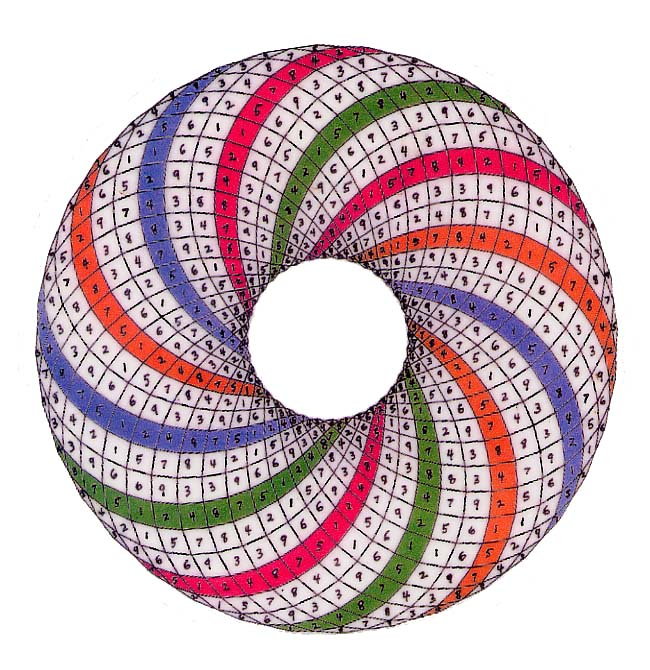And the 9 demonstrates the omni dimension which is the higher dimensional flux emanation called Spirit that always occurs within the center of the magnetic field lines. The last number left to be explained from TheMATHEMATICAL FINGER PRINT OF GOD is the number 9. The number nine is Energy being manifested in a single moment event of occurrence in our physical world of creation. It is unique because it is the focal center by being the only number identifying with the vertical upright axis. It is the singularity or the Primal Point of Unity. The number nine never changes and is linear. For example all multiples of 9 equal 9. 9x1=9, 9x2=18, but 1+8=9, 9x3=27, but 2+7=9. This is because it is emanating in a straight line from the center of mass out of the nucleus of every atom, and from out of the singularity of a black hole. It is complete, revealing perfection, and has no parity because it always equals itself. The number nine is the missing particle in the universe known as Dark Matter.

The number nine lines up with the center of the infinity symbol and it is from this center that the linear emanations we call Spirit emanate from the center of mass outwards. Spirit is the only thing in the universe that moves in a straight line. Spirit is the inertia aether that Einstein postulated. Spirit is what makes everything else warp and curve around it. The perfect number patterns are actually created by this Spirit energy. Without Spirit the universe would become destitute and void. Spirit flow is the source of all movement as well as the source of the non-decaying spin of the electron.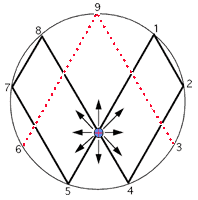Numbers have temporal, spatial and volumetric qualities. For example, physics is the base ten number system. In other words numbers have bilateral or mirror symmetry and line up in stratified layers of horizontal planes. This is the same as physics' parity. There are nine simple multiplication series (one series for each discrete number 1-9) in Vortex-Based Mathematics. These multiplication series are based upon reducing all products to single digits via horizontal addition forming meaningful number sequences.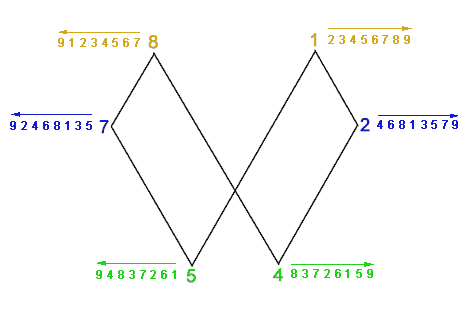There are three polar number pairs; 1 and 8, 2 and 7, 4 and 5. For example, multiples of 4 are 1x4=4, 2x4=8, 3x4=12 but 1+2=3, 4x4=16 but 1+6=7, 5x4=20 but 2+0=2, 6x4=24 but 2+4=6, 7x4=28 but 2+8=10 and 1+0=1, 8x4=32 but 3+2=5, 9x4=36 but 3+6=9. So multiples of 4 equal 4,8,3,7,2,6,1,5 and multiples of 5, the polar opposite of 4, are (5,1,6,2,7,3,8,4). Each pair forms number sequences that are identical but in opposing directions. So if you take the multiples of 5 and look at them running backwards they are the same as the multiples of 4 running forwards. In exactly the same way the multiplication series for the polar pair [1,8] is: (1,2,3,4,5,6,7,8) and (8,7,6,5,4,3,2,1) for the number 1 and the number 8 respectively. The multiplication series for the polar pair [2,7] is: (2,4,6,8,1,3,5,7) and (7,5,3,1,8,6,4,2). Everything is starting from the center outwards, from the singularity.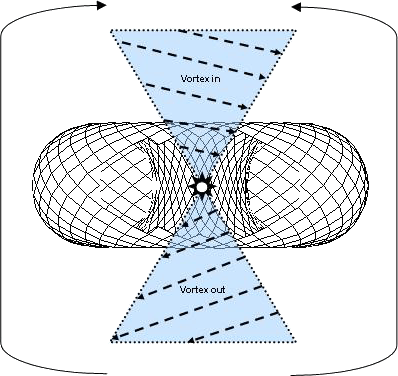Nature is expressing herself with numbers. The symmetry of our decimal system is a principle of nature. The 9 axis causes the doubling circuit and it is the point towards which matter converges and away from which it diverges or expands. Thus the polar number pairs will be mirror images of each other, both flowing in opposite directions from the central axis. There is perfect symmetry wrapped around a single point coiling outwards the way that petals are wrapped in a rose, or a nautilus shell spirals outward.

We ARE emanations of this numerical pattern, of this vortexual expression and it's time to use this to our distinct advantage, energetically, technologically, and spiritually.

Source: http://rense.com/rodinaerodynamics.htm

Source:

http://energyinmotionearthtrek.blogspot.com/2014/01/mathematical-fingerprint-of-universe.html

1 comment :

1.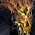Marko Rodin and his "Rodin" coil has been known for some years on the internet and you-tube but it's good to see it get more recognition. This can also be seen in a youtube video titled; Ancient knowledge pt. 6-1 number 9 code, vortex based math. These people need funding and that's their problem despite so many keep telling us we don't need a money based economy we s are still in the middle of using one.

SITS blog is a venue where Data we come across can be shared with all of you. If we look past personal bias, and distill the Absolute Data within each post, our natural intuition will assemble these nuggets together and reveal a greater truth.

We do not know what that truth is yet of course. We are discovering that together as a whole by sharing and discussing our unique perspective. Share your thoughts and we will all come to a greater understanding as one.

Support Stillness in the Storm